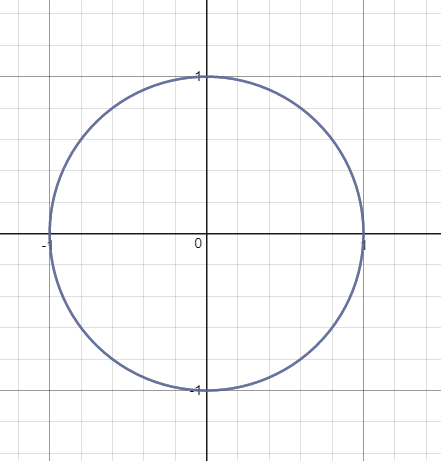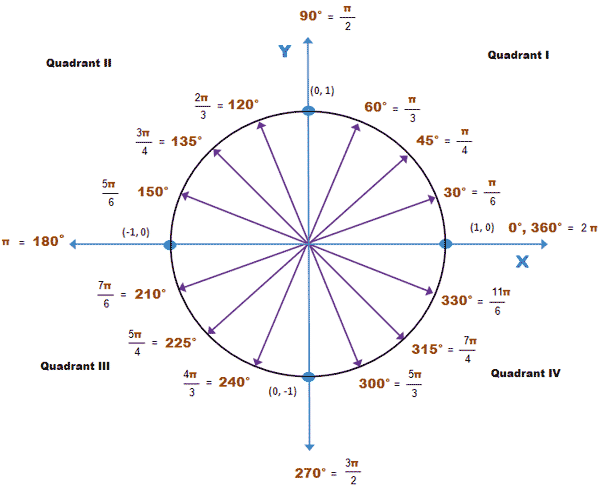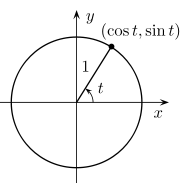# Make revolving parts around a character

Hey all,

I am trying to make a system that can revolve any amount of parts around a character, while keeping the same spacing between parts.

Here is an image of what I am trying to accomplish (notice the motor is always facing the character, I am trying to keep the front of the part always facing the character)

That is an image using 8 bricks, here is how it would look at 4 bricks.

I am confused as to where to start here, how to position them according to the character, and how to revolve them in a circle.

My first guess would be to use the number 360, and divide it by the amount of parts, then multiply.

Like this:

``````local spacing = 360/(#game.Workspace.Player.Parts:GetChildren())
part.Orientation = spacing * part_num
``````

That would work for the rotation great! But now how to I apply it so it moves around the player in a circle like so?

26 Likes

Could you place an Attachment and a HingeConstraint in the player and put Attachments in each Part to that?
Dunno how a HingeConstraint would work with something as mobile as a Player, but they seem pretty stable on vehicles.
You’d probably have to check the Massless property of all the Parts that are rotating so they don’t affect the Player’s movements.

1 Like

Yeah that would work, I am probably going to just update the positions on movement though.

The thing I am having trouble with is actually positioning the parts around in a circle.

1 Like

So to start off, you probably need to know what the unit circle is in order to do this. Since you’re really only working in two dimensions (the height of the parts remains constant), we can represent your problem with x and y on a plane like so:You can go here to play around with it:

An important thing to remember is that the unit circle uses radians, not degrees. Instead of representing a point on this circle with an x and y, it’d be much more intuitive to use a single angle measurement, right?

The full distance around the circle in radians comes out to be 2 * pi, here’s a chart for other measurements around the circle:Since a full rotation is 2 * pi radians, a half rotation is just pi, or (2 * pi) / 2. Using this way of thinking, one can reason that (2 * pi) / an amount gives you the radian measurement for that length around the circle. Say you wanted 1/8 of the circle, well then (2 * pi) / 8 would give you that.

If we think about doing this in lua the easiest way would be to set up a table of parts that you would want to manipulate. Since this is part of the character, I’ll put the script in StarterCharacterScripts:

``````local character = script.Parent
local hrp = character:WaitForChild("HumanoidRootPart")

local parts = {}
local numberOfParts = 8

for _ = 1, numberOfParts do
table.insert(parts, Instance.new("Part")) -- put the parts into the table
end

for i, part in pairs(parts) do -- set the properties of the parts
part.Anchored = true
part.CanCollide = false
part.Parent = workspace
end
``````

Then we can set up some variables for the unit circle

``````local fullCircle = 2 * math.pi
local radius = 10 -- the radius of the parts from the character
``````

Now we need to figure out how we would get the x and y coordinates from the angle, luckily for us mathematicians have worked this out already, the y on a unit circle can be found by sin(angle) and the x can be found by cos(angle):
(t being the angle in radians)We can write up a function to do this to get the x and y positions for any given angle, but since we’re not changing the y position of the part, only the x and z, we’ll use z instead of y. We can also multiply each number by the radius to set the radius of the circle, like this:

``````local function getXAndZPositions(angle)
local x = math.cos(angle) * radius
local z = math.sin(angle) * radius
return x, z
end
``````

I think the best way to set the positions is to set the positions every frame, but you might think of a better way. Here’s what I’ve done with a function connected to Heartbeat in RunService.

To get the angle of the part, you can get the section of the circle for the first part and then multiply it by the number of the current part

``````game:GetService("RunService").Heartbeat:Connect(function()
for i, part in pairs(parts) do
local angle = i * (fullCircle / #parts)
local x, z = getXAndZPositions(angle)

-- multiplying CFrames combines them:
local position = (hrp.CFrame * CFrame.new(x, 0, z)).p -- .p gets the position part of the CFrame as a Vector3
local lookAt = hrp.Position

part.CFrame = CFrame.new(position, lookAt) -- a CFrame constructor that takes a position and a lookAt value and returns a CFrame looking that that position
end
end)
``````

At the end of it all, you get this!

Here’s all my code in one place:

``````local character = script.Parent
local hrp = character:WaitForChild("HumanoidRootPart")

local parts = {}
local numberOfParts = 8

for _ = 1, numberOfParts do
table.insert(parts, Instance.new("Part"))
end

for i, part in pairs(parts) do
part.Anchored = true
part.CanCollide = false
part.Parent = workspace
end

local fullCircle = 2 * math.pi

local function getXAndZPositions(angle)
local x = math.cos(angle) * radius
local z = math.sin(angle) * radius
return x, z
end

game:GetService("RunService").Heartbeat:Connect(function()
for i, part in pairs(parts) do
local angle = i * (fullCircle / #parts)
local x, z = getXAndZPositions(angle)

local position = (hrp.CFrame * CFrame.new(x, 0, z)).p
local lookAt = hrp.Position

part.CFrame = CFrame.new(position, lookAt)
end
end)
``````
187 Likes

That is an awesome tutorial thank you so much! You helped me out a ton!

6 Likes

is there any way to make the parts stop moving and revolving around you and just stay in place?

Don’t update the cframes every Heartbeat

1 Like

this isn’t gonna crash your game but personally I wouldn’t do this over a period of time.

That wasn’t the reason for my message. I was answering the question above.

oh the question above you doesn’t say anything about that didn’t know sorry

How can I do a 3d version of it? I made a linear version on the Y vector but its forming a losang, it would be cool to have it round

2 Likes